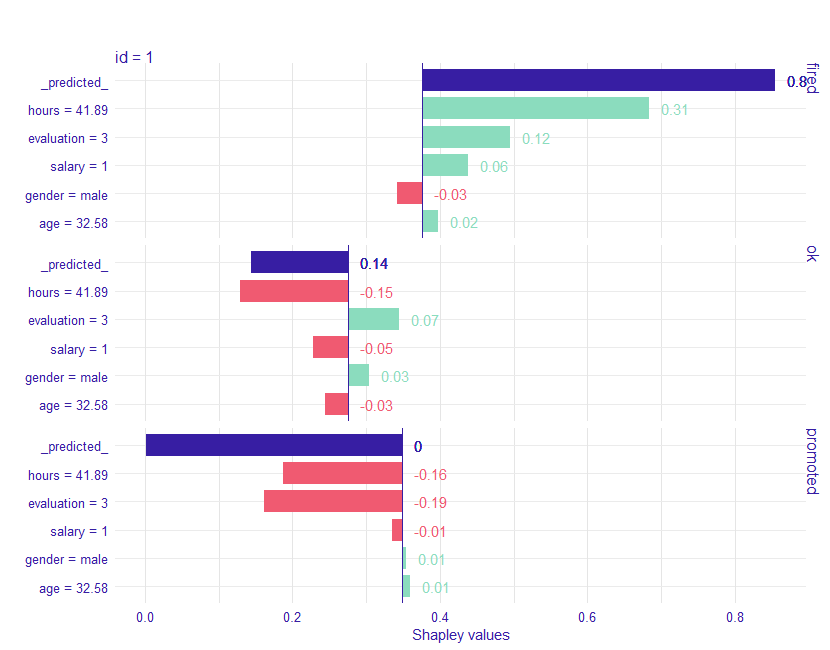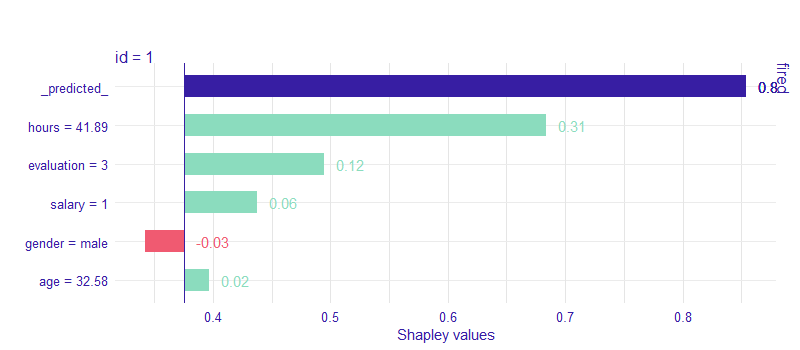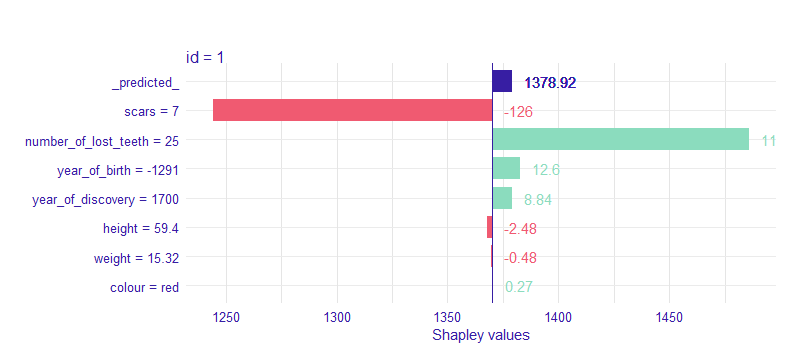An R wrapper of SHAP python library

Pkgdown Website

Blog post with gentle introduction to shapper

## Installation and configuration

Install shapper R package

devtools::install_github("ModelOriented/shapper")

You can install shap Python library via

shapper::install_shap()

If installation didn’t work for some reason. Try installing dependencies first

reticulate::py_install(c("numpy", "pandas"))

or

reticulate::conda_install(c("numpy", "pandas"))

Python library SHAP can be also installed from PyPI

pip install shap

or conda-forge

conda install -c conda-forge shap

For more details how to configure python paths and environments for R see reticulate.

## Classification Example

# instal shapper
# devtools::install_github("ModelOriented/shapper")

# install shap python library
# shapper::install_shap()

# devtools::install_github("ModelOriented/DALEX2")
library("DALEX2")
Y_train <- HR$status x_train <- HR[ , -6] # Let's build models library("randomForest") set.seed(123) model_rf <- randomForest(x = x_train, y = Y_train) # here shapper starts # load shapper library(shapper) p_function <- function(model, data) predict(model, newdata = data, type = "prob") ive_rf <- individual_variable_effect(model_rf, data = x_train, predict_function = p_function, new_observation = x_train[1:2,], nsamples = 50) # plot plot(ive_rf)# filtered ive_rf_filtered <- dplyr::filter(ive_rf, _ylevel_ =="fired") shapper:::plot.individual_variable_effect(ive_rf_filtered)# Regression example library(shapper) library("DALEX2") library("randomForest") Y_train <- dragons$life_length
x_train <- dragons[ , -8]

set.seed(123)
model_rf <- randomForest(x = x_train, y = Y_train)

ive_rf <- individual_variable_effect(model_rf, data = x_train,
new_observation = x_train[1,])

plot(ive_rf)